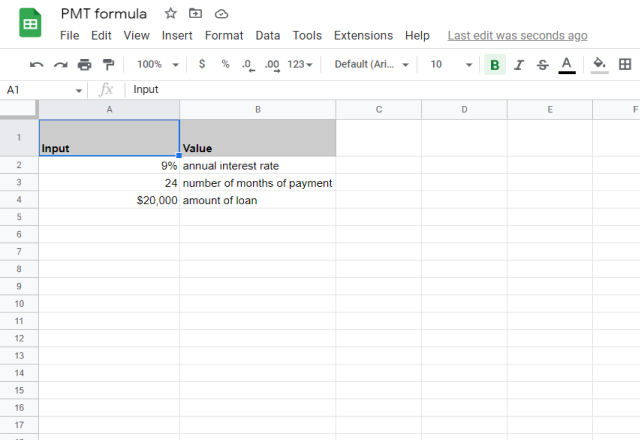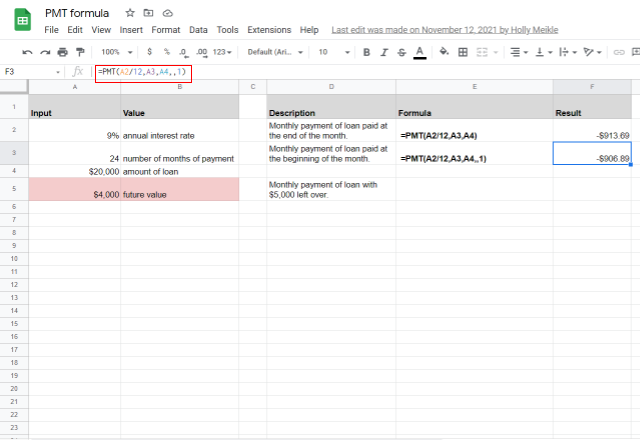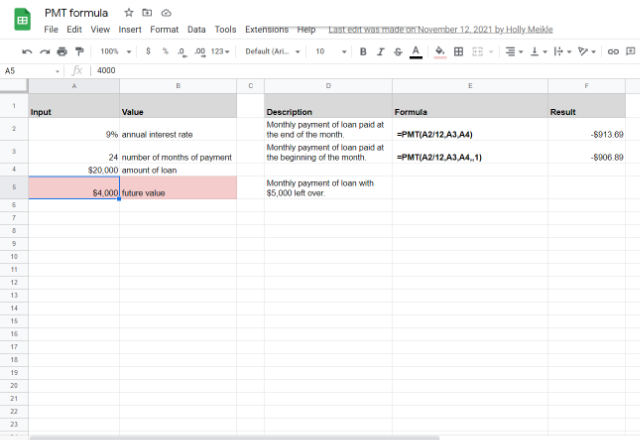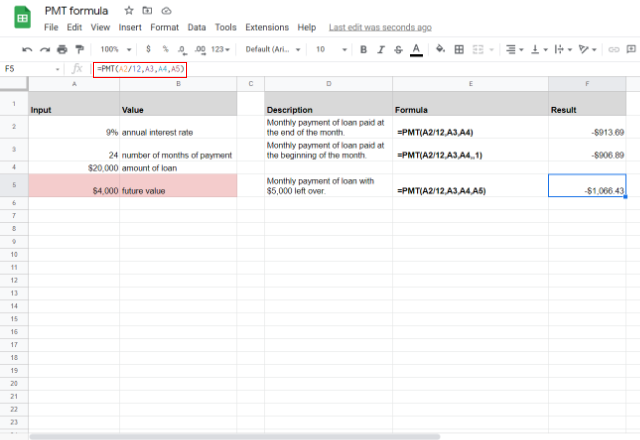# How to use the PMT formula in Google SheetsNowadays, long-term payments are becoming more and more popular in both personal and professional environments. Suppose you need to pay back a loan from an initial business investment, or you are investing parts of your income on a regular basis. It’s wise to have a good idea of the final amount you’ll pay at the end of the payment period. Rather than spending time carrying out complex calculations, we can use the PMT formula in Google Sheets to calculate everything for us.

## Why use the PMT formula?

When it comes to finances, it’s extremely important to get everything right. By using the PMT formula, not only are you avoiding any risk of human error to receive an accurate result, but you can also have a clear understanding of your payments long-term. The PMT works in any long-term payment situation, whether it’s for 30 months or 30 years.

Here are just a few examples of when you’d use the PMT formula:

• Car loan
• Mortgage
• Student loans

## What is the PMT formula?

The PMT formula is used to calculate constant payments that have a constant interest rate. You can calculate the payments for the loan via the given loan amount, interest rate, and the number of payments.

### Syntax

Here’s the following PMT formula:

=PMT(rate, number_of_periods, present_value, [future_value, end_or_beginning])
• rate – is the rate of interest.
• number_of_periods – is the number of periods that the buyer wishes to make payment for.
• present_value – is the current value of the annuity.
• future_value – OPTIONAL: is the amount of the future value that remains after we make the final payment.
• end_or_beginning – OPTIONAL (0 by default): a 0 indicates that we are making the payments at the end of each period. And a value of 1 specifies that we are making payments at the beginning of each payment period.

• In order for the formula to work, the interest rate and time period between installments (months, years, quarters…) must be fixed.
• The formula does not take into account other aspects such as tax or other fees.
• You must keep the units consistent throughout the entire formula.
• > If you’re paying a monthly payment for 4 years, then your number_of_periods value will be 12*4.
> If your installments are monthly, then you must divide the annual interest rate by 12 to get the ‘monthly’ rate.

Now that we understand what the PMT formula is and what it can be used for, let’s explore how to use the PMT formula in Google Sheets.

### How to use the PMT formula in Google Sheets

Let’s say I am an entrepreneur who has just started my new business. I have taken a loan out of \$20,000, with an annual interest rate of 9%.

I am paying back in monthly installments over two years – 24 periods.Using the PMT formula, I can calculate exactly how much I will have to pay back each month.

In order to calculate the amount I will have to pay at the end of every month, the PMT formula should look like this:

=PMT(A2/12,A3,A4)

Here is my result. I will have to pay \$913.69 every month to pay back my loan (with interest) over 2 years.Now let’s say I want to pay my loan at the beginning of every month. The PMT formula will look like this:

=PMT(A2/12,A3,A4,,1)
Please note: As we haven’t inputted the future_value value, we need to add 2 commas to keep the calculation accurate.

Here is my result. My monthly payment reduces to \$906.89 per month.Now let’s say I want a balance of \$5,000 leftover from my last monthly payment.The PMT formula will then look like this:

=PMT(A2/12,A3,A4,A5)

Here is my result. The monthly payment is larger at \$1066.43.#### The PPMT and IPMT formulas

There are 2 other formulas that are closely linked to the PMT formula, in order to help us learn more about our loans or investments:

• PPMT: This function calculates the principal value of your payments over a particular period.
• IPMT: This function calculates the interest value of your payments over a particular period.

In short, these two formulas can help to calculate the two aspects of your monthly payments (principal and interest) that have been calculated using the PMT.